# Neural Network based Diagnostic of PEM Fuel Cell

Neural Network based Diagnostic of PEM Fuel Cell

Laboratoire de Modélisation des Systèmes Energétiques «LMSE», University of Mohamed Kheider, BP145, Biskra 7000, Algeria

Laboratoire de Recherche en Electronique de Skikda, Université 20 Août Skikda, BP 26 Route d'El-hadaeik, Skikda 21000, Algeria

Laboratoire d'Analyse des Signaux et Systémes (LASS), Department of Electronics, University of M'sila, BP 166, Route Ichebilia, M'sila 28000, Algeria

Corresponding Author Email:
hicham.kahia@univ-biskra.dz
Page:
225-234
|
DOI:
https://doi.org/10.14447/jnmes.v23i4.a02
1 September 2020
|
Accepted:
26 October 2020
|
Published:
31 December 2020
| Citation
Abstract:

This paper focuses in finding a suitable, effective, and easy to use method, to avoid the frequent mistakes that are presented by the poor flow of water inside the fuel cell during its operation. Towards this aim, the artificial intelligence technology is proposed. More specifically, a neural network model is used to enable monitoring the influence of the humidity content of the fuel cell membrane, through employing electrochemical impedance spectroscopy method (EIS analysis). This technique allows analyzing and diagnosing PEM fuel cell failure modes (flooding & drying). The benefit of this work is summed up in the demonstration of the existence in a simple way that helps to define the state of health of the PEMFC.

Keywords:

PEMFC, neural network, EIS

1. Introduction

No alternative fuel today meets public acceptance for competing with traditional fossil fuels, which have benefited from nearly a century of continuous improvement by the oil industry. Currently, the energy crisis and the increase in the level of pollution are major problems worldwide. New renewable and clean energy sources must, therefore, be considered and this will be one of the eminent challenges in these years, both economically and environmentally [1-2].

Soon, fuel cell technology will be considered as a renewable primary energy source. The fuel cell will generate electrical energy from hydrogen; this is why it has become one of the key energy converters for the future, whether for stationary and on-board applications (laptops, cars, buses, planes, scooters, boats, and submarines) .

There are various types of fuel cells which are classified based on the nature of the electrolyte and/or the operating temperature. Among these categories of the fuel cell, we find PEM (Polymer Exchange Membrane) fuel cell. The electrolyte (i.e. polymer membrane) allows the transport of protons to the cathode side from the anode side. The electrons, meanwhile, move inside external load, thereby producing useful electrical power. The overall electrochemical reaction occurring throughout the PEMFC is defined as follows:

O2 + 2H2→ 2H2O  + Electrical energy + Heat       (1)

The enthalpy energy of the reaction can be calculated using Hess's law Eq. (2). Under standard conditions of pressure and temperature (1Bar and 298K or 25 ° C):

$\Delta r H=\Sigma v i \Delta H i$       (2)

Currently, many researchers revolve around augmenting the service life and understanding the aging mechanisms in a fuel cell system, e.g. modifying air flow rate, humidifying gases, etc. to confirm the proper functioning of the PEMFC. Therefore, the diagnosis of PEMFC (i.e. the technique of detection and identification of faults) are treated essentially in the literature, for instance, the works in [4-8].

The fuel cell must operate under conditions where the speed of evaporation and evacuation of water is slower than its production’s speed to keep the membrane hydrated. Some operating conditions applied to the fuel cell may lead to produce two types of antagonistic faults (drowning and drying) as displayed in Figure 1. Generally, the detected faults are linked to the management of water in the membrane [10-13]. Among the most used methods for characterization is electrochemical impedance spectroscopy (EIS). This method is employed to measure the value of the electrical resistance or for monitoring degradation [14–18]. During each degradation phase, EIS can be carried out to characterize the impedance and describe its evolution of the parameters in order to allow differencing between drying and flooding.

There are a significant amount of methodological approaches to identify and diagnose a fuel cell system, which can be classified into five families, i.e. semi-empirical models, knowledge models, black-box models, empirical approaches, and finally information processing techniques. These methodologies can be normally divided into two groups: so-called static methods or so-called dynamic methods. The static approach is particularly interesting to make a technological choice concerning like the catalyst or to size components of the cell). While, dynamic methods are preferable when one wishes to analyze transient phenomena such as a sudden change of a set point or a parameter.

## 1.jpg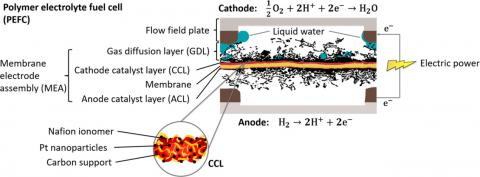Figure 1. Diagram of the PEMFC components in operation, received from the visualization of XCT.

Many researchers have proposed the use of neural network method to diagnosis the PEM fuel cells [10, 19-22]. For example, the authors in  studied the methodology of a dynamic neural network to control the process of a system (PEMFC). The objective of their work is to follow the variations imposed on the system, starting with the introduction of a delay line at the input of the (NNT) to obtain a dynamic (NNT) control model. Steiner et al.  proposed a model using (NNT) and they demonstrated the difference between the normal functioning deliberate by a neural network and the real functioning, in order to classify the health states (SOH) of the fuel cells (flood, normal functioning, and drying out). Jonghoon et al.  explored diagnostic approach employing pattern recognition model identification (FCOV) based on Hamming NNT application. to select the value of (ΔRd) of the cell, and then use it to diagnose the state of a cell. While, Cadet et al.  have proposed indicators allowing evaluating the diagnostic performances, from giving specific equations to assess the degree of cell's humidity. In order to model power supply purpose for an embedded system of (PEMFC), Jeme et al.  have designed a neural network model that it will be possible to carry out developments to control the transfer of energy in a fuel cell vehicle Meng Shao et al.  have designed the PEMFC dynamic model, which is built and simulated using MATLAB. In this work, the ANN ensemble for the fault diagnosis (i.e., Fault in the stack cooling system; Increasing of fuel crossover; Fault in air delivery system; Fault in hydrogen delivery diagnosis) is built to improve the stability and reliability of the PEMFC systems.  The authors in  studied the technique to predict water activity in (PEMFC) from the (EIS) and adapted a neuro-fuzzy inference system (ANFIS) as an estimator. Slimane Laribia et al.  have defined and implemented a method employing an artificial neural network to create an optimal impedance model of the (PEMFC), which respects the mass transfer theory, across the physical parameters of the (EIS) model. Based on their ANN model, the authors have diagnosed PEM fuel cell failure modes (flooding & drying). For other works on diagnostic of PEMFC using EIS method, the reader is encouraged to refer to [17,34-36].

Since the development of control systems in industrial applications must minimize the number of instruments for simple methodological diagnosis, in this study, we use a black box model based on neural network, because it is easy and quick to implement. More specifically, this work aims to determine the state of the electrochemical response during the use of the fuel cell through designing a neural network-based strategy for the diagnostic of PEM fuel cell to fulfill the efficiency needs.

The contributions of our work can be summarized as follows

- A fast neural network-based model to diagnosis of PEM fuel cell.

- The proposed model could be useful for industrial applications.

The rest of this paper is organized as follows. Section 2 discusses the principle features of fuel cell. Section 3 presents the proposed controller model using neural network for diagnostic of PEMFC. Section 4 provides the obtained results. Finally, the paper is concluded in section 5.

2. Inciple Features of Fuel Cell

2.1 Structure of the PEMFC system

Figure 2 shows the operation of a fuel cell core, which requires a large number of auxiliaries essential for a proper functioning. The overall system is called the fuel cell system, that comprises the cell core and the auxiliaries (i.e. the hydrogen system which supplies the anode with hydrogen gas, the air system provides the cathode with oxygen, The key role of the valve is to regulate the pressure in the two systems (i.e., Air compressor and Hydrogen source) through the pressure sensor. Whilst, the humidifier and the cooling system maintain the humidity level and the temperature of the fuel cell, respectively) . The behavior of the stack is strongly influenced by that of the auxiliaries, for instance, the humidification subsystem (drying or over-humidification of the membrane) reduces the production of electrical energy and limits the life of the PEMFC.

## 2.jpgFigure 2. Structure of a PEM fuel cell system. 

2.2 Model of PEM fuel cell

The model of the assembly selected is that presented by JC Amphlett et al [add reference], this electrochemical model can be used to describe the dynamic behavior of an assembly of the PEMFC; it makes it possible to take into account the different parameters that are essential for a good result.

At the level of an elementary cell, the quasi-static model is used to predict the voltage response of the cell as a function of current, temperature, partial pressures of the reactive gases, and the hydration of the membrane. The real potential of a PEMFC is decreased in comparison with the Nernst potential due to the polarizations such as activation, ohmic, and concentration. This express is given as   [23- 25]:

Vcell = ENerstVactVohm Vcon                     (3)

The thermodynamic potential is defined by a Nernst equation in developed form as , , :

$E_{n e r s t}=1,229-0,85.10^{-3}(T-298,15)+$$4,31.10^{-5} . T .\left[\operatorname{Ln}\left(P_{H 2}\right)+\frac{1}{2} \operatorname{Ln}\left(P_{O 2}\right)\right] (4) Where T denotes the cell temperature [K]. PH2 and PO2 are partial hydrogen and oxygen pressures [atm]. Note that the activation polarization at the anode increases with the current density, its expression can be written in the form , , , : V_{a c t}=\xi_{1}+\xi_{2} \cdot T+\xi_{3} \cdot T \cdot \ln \ln \left(C_{O 2}\right)+\xi_{4} \ln \ln \left(I_{\text {stack }}\right) (5) Where: I stack are the operating current of the fuel cell (A), and ξ1; ξ 2; ξ 3; ξ 4 are the parametric coefficients appropriate to each fuel cell model, Table 1 identifies the exact parametric coefficients used in our model, T are the temperature of fuel cell. CO2 is the concentration of oxygen in the catalytic interface. It is expressed by Henry's law as follows: C_{O 2}=\frac{P_{O 2}}{5,08.10^{6} \cdot e^{\left(\frac{-498}{T)}\right.}} (6) The losses of the concentration polarization are given by the following relation: V_{c o n}=-B \cdot \ln \left(1-\frac{J}{J \max }\right) (7) Where B is an empirical constant that depends on the type of FC and its operating state , . J is the current density of the permanent operation; Jmax is the maximum current density. The electrolyte and the electrodes obey Ohm's law. We can express the ohmic losses by the following equation: V_{\text {ohmic }}=I_{\text {stack }}\left(\frac{t_{m}}{\sigma_{m}}+R_{C}\right) (8) Steam diffusion coefficient of water vapor in the membrane is calculated by, : \sigma_{m}=(0,00519 \lambda-0,00324) \exp \exp \left(1268\left(\frac{1}{303}-\right.\right.$$\left.\left.\frac{1}{T_{f c}(K)}\right)\right)$  (9)

The water content of the membrane is presented as:

$\lambda=\left\{0,043+17,81 \phi-39,85 \phi^{2}+36 \phi^{3}, 0 \leq \phi\right.\leq 114$

$\quad+1,4(\phi-1), \quad 1 \leq \phi\leq 3$             (10)

The problem of water management is to maintain a constant coefficient of hydration of the membrane. The latter is sensitive to drying and flooding ; these two constraints slow the speed of gas passage and degrade the membrane. Therefore, it is very important to follow the state of relative humidity to keep the membrane properly hydrated and given as , , .

$\varphi=\frac{P_{\text {wout }}}{P_{\text {sat }}}$        (11)

The water vapour partial pressure Pwout is related to the absolute pressure at the output of the stack Pexit (atm), as represented by the following equation:

$P_{\text {wout }}=\frac{(0,42+\lambda \psi) P_{\text {exit }}}{\lambda(1+\psi)+0,21}$         (12)

The saturated vapour pressure Psat(atm) depends on the temperature, which is given by the following relation.

$P_{\text {sat }}=10^{5} \exp \exp \left(13,7-\left(\frac{5120}{T_{\text {air }}+273,15}\right)\right)$    (13)

ψ is calculated by:

$\psi=\left(\frac{q_{\text {win }}}{q_{\text {O2in }}+q_{\text {rest }}}\right)$    (14)

$\left\{q_{\text {O2in }}=\frac{\lambda . I_{\text {stack }}}{4 . F} q_{\text {rest }}=3,76 \frac{\lambda\text { .Istack }}{4 . F}\right.$       (15)

qwin: molar flow air in the inlet.

qO2in: the molar oxygen flow rate at the inlet.

qrest: the molar flow rate of non-oxygen (N2) in the air.

The humidity of the membrane is affected in several parameters like humid airflow qwin.

## 3.jpgFigure 3.Relative humidity (%) curve as a function of the water flow rate input in PEMFC.

Figure 3 shows the change of humidity when changing qwin and its relationship to the state of PEMFC when the temperature is constant. During the change of the value of qwin, we can notice that there are three divided regions according to the percentage of relative humidity:

- qwin < 0.4*10-5 : too dry.

- qwin > 1.5*10-5: too wet.

To make fuel cell operates in normal conditions, the humid air flow must be between these two mentioned values.

## 4.jpg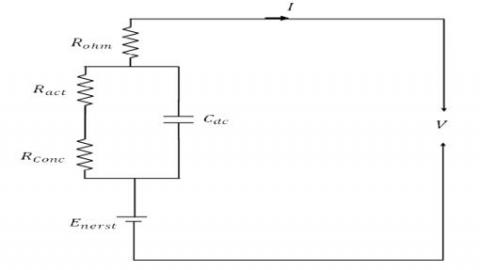Figure 4. Simplified dynamic model

Figure 4 presents PEMFC in the form of an electrical circuit in a simple structure i.e. a voltage source corresponding to the Nernst potential in series with some resistances representing the voltage drops. Also, the fluid dynamics have been taken into account through using equations (16-19), involving the partial pressure of hydrogen (respective oxygen) and the flow of hydrogen entering the cell (respective oxygen).

The global dynamic models help to make it possible to predict the electrical response (voltage or current) of the stack .

$P_{H 2}=\frac{\frac{1}{K_{H 2}}}{1+\tau_{H 2} \cdot S}\left(q_{H 2}-2 K_{r} I_{\text {stack }}\right)$            (16)

$\tau_{H 2}=\frac{V_{a n}}{R \cdot T \cdot K_{H 2}}$         (17)

$P_{O 2}=\frac{\frac{1}{K_{O 2}}}{1+\tau_{O 2} \cdot S}\left(q_{O 2 i n}-2 K_{r} I_{\text {stack }}\right)$        (18)

$\tau_{O 2}=\frac{V_{a n}}{R \cdot T \cdot K_{O 2}}$        (19)

KH2: gain of the hydrogen flow.

KO2: gain of the oxygen flow.

τH2: time constant of the hydrogen flow.

τO2: time constant of the oxygen flow.

2.3 Modeling of the PEMFC impedance model

Electrochemical Impedance Spectroscopy is a widely used method for fundamental analysis of existing phenomena in electrochemical devices. In a PEMFC, the equivalent impedances are used to determine the electrochemical parameters (double layer capacity, resistances of the membrane and connections, charge transfer resistance, etc.) or to analyze the internal behavior of the PEMFC (influence of the humidification and drying of the membranes , monitoring of the lifetime of the PEMFC . The most commonly used equivalent circuit in measurement models is the Randles circuit . Figure 5 shows an equivalent Stack of PEMFC model, the fuel cell impedance is expressed as:

$Z_{c e l l}(j w)=R_{m}+\frac{1}{Z_{C P E}+\left(\frac{1}{\left.\left(R_{P}+Z_{W}\right)\right)}\right.}$     (20)

The Warburg impedance is defined as follows , , :

$Z_{W}(j w)=R_{d} \frac{\tanh \sqrt{\left(j w\left(\tau_{d}\right)\right)}}{\sqrt{j w \tau_{d}}}$        (21)

## 5.jpg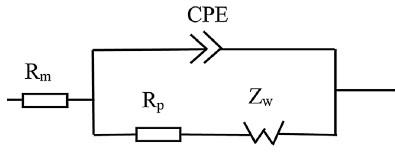Figure 5. Randles cell with CPE impedance.

The constant (τd), (Rd) and ZCPE are given as follows:

$\left\{\tau_{d}=\frac{\delta^{2}}{D} R_{d}=\frac{R T \delta}{n^{2} F^{2} S C D} Z_{C P E}(j w)=\frac{1}{Q(j w)^{\alpha}}\right.$         (22)

Therefore, the equivalent PEMFC impedance is calculated by the following equation:

$Z_{T}(j w)=R_{m}+\frac{1}{Q(j w)^{\alpha}+\left(\frac{1}{\left.\left(R_{P}+Z_{W}\right)\right)}\right.}$     (23)

If α = 1, CPE is an ideal capacity.

When α = 0.5, CPE is the Warburg impedance.

Figure 6. illustrates spectroscopy impedance for a fuel cell at a different state of PEMFC, presented by (CPE) model and confirmed by experimental data for ,. In the normal state, we have a small arc in the low and high frequency, while in the case of drowning we notice that there is a large arc, unlike the drought state we observe the withdrawal of the arc presented in the Nyquist plot on the right side. Depending on these observations, we can find the state of the fuel cell by studying the parameters of Rm, Rp, and Rd (equation (23)). More details about these parameters are given subsection 3.2.

## 6.jpg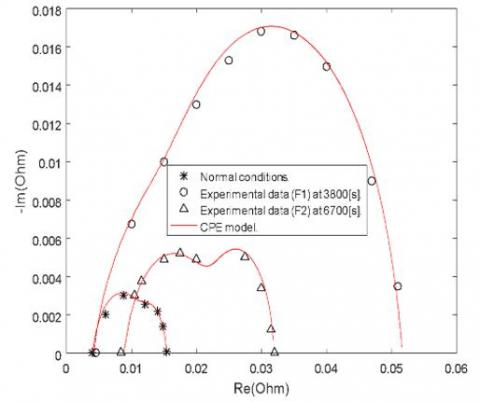Figure 6. The Nyquist plot of the fuel cell impedance

3. Controller Model by NNT for Diagnostic PEMFC

Neural networks are experiencing a resurgence of interest and even a huge hype under the name of deep learning. An artificial neural network is a system inspired by the functioning of the human brain to learn. Typically, the artificial neural network used to receive relationships between inputs and outputs in a neural network model developed for a certain application. The weights can be changed by the transfer function to ensure the connection between the three layers of neuron network model using a well-defined training rule. In order to train the NNT, its parameters such as the architecture, the data, and activation function should be well defined. This phase is very important since it represents an iterative procedure of estimating the parameters of network neurons in order for the latter to fulfill its task. Once the training phase is completed, the trained network model must be able to make the right decision for input vectors that it has not learned. Here, the NNT training performance is estimated using the Mean Squared Error (MSE); the MSE is computed by comparing the values of the NNT output and the desired value that used as a tool for updating the weights utilizing the dynamic gradient descent algorithm.

To diagnose state of fuel cell health (PEMFC) using NNT model, the water flow rate input (qwin) and operating time (t) are fed to the NNT. Based on the outputs of NNT model, which are the values of the physical parameters of the EIS model (Rm, Rp, and Rd), one can diagnose the state of the fuel cell. Finally, the attained results by NNT model are compared with those obtained experimentally by Fouquet et al. .

3.1. PEMFC and ANN Architecture

The sub-models of the fuel cell system (pressure, voltage and relative humidity calculator) are presented in Figure 7, whilst, the parameters of this model presented in Table 1.

Table 1. Parameters of a normal operating condition of the PEMFC model.

 Parameters Value curent density : Jn 0.35 [A/cm2] Temperature: T 320 [K] Active area : S 200 [cm2] Aair stoichimetry :λa 3 Nember of fuel cells :N0 10 ζ1 −0.948 ζ2 0.00286+0.0002.lnS+  (4.3.10−5) lnCH2 ζ3 7.6 × 10−5 ζ4 −1.93 × 10−4 Hydrogen valve constant : KH2 4.22 × 10−5[kmol]/[s.A] Oxygen valve constant : KO2 2.11 × 10−5[ kmol]/[s.atm] Hydrogen time constant: τH2 3.37 [s] Oxygen time constant : τO2 6.74 [s] Hydrogen –Oxygen flow ratio : rH-O 1.168 Kr constant =N0/4F 0.996×10−6[kmol]/[s.A] Vn(cell) 0.72 [V] Water flow molare :qwin [0-3] ×10-4[mole/s]

The multilayer perceptron is a network made up of successive layers of neurons. An input layer reads the incoming signals; an output layer provides the system’s response. Besides, one or more hidden layers participate in the transfer of information. In a perceptron, a neuron from a hidden layer is connected as an input to each of the neurons of the previous layer and as output to each neuron of the next layer.

## 7.jpg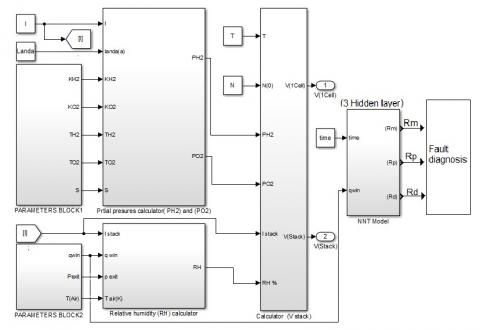Figure 7.PEMFC and ANN block diagram.

The transfer function used in the hidden layer is defined by the following equation:

$f(u)=\frac{1}{1+e^{-(d . u)}}$     (24)

Where u represents the input of the hidden layer and calculated as:

$u=\sum_{j=1}^{n} \left(W_{i j} X_{i}+b_{i}\right)$  (25)

For the output layer, the equation that represents the network model is expressed as

$y_{K} u=\sum_{j=1}^{N} \left(w_{i j}^{0} u_{i}+b_{i}\right)$$=\sum_{j=1}^{N} \operatorname{} w_{i j}^{0} f\left(\sum_{j=1}^{N} \left(w_{i j} x_{i}+b_{i}\right)\right)$           (26)

Where yk indicates the output signal from kth output neuron, w0ki represents the weight of ith output ui to the kth neuron in the output layer and ui is the activation value of the jth neuron in the ith layer.

The following two choices (the number of hidden layers and the number of neurons per hidden layer) directly condition the number of parameters (weight W) to estimate the complexity of the model; they participate in the search for a good compromise (bias/variance), i.e. the balance between quality of learning and quality of forecasting. The choice mainly concerns the control of over-learning limits the number of neurons or the learning time or even increasing the penalization coefficient of the parameter norm. This requires determining a method for estimating the error, validation or test sample, cross-validation, or rap boots.

3.2. Neural network-based controller model

To simulate Matlab how humidity affects the state of the fuel cell and how to change the values of the Rm, Rp, and Rd when it is operating during periods, we formed a model of a neural network as shown in Figure 7 that is constituted  of two inputs qwin and t, in addition, three outputs representing the values of Rm, Rp, and Rd to express the state of the fuel cell, three hidden layers for each contain (10, 5, 5) neuron, respectively. Besides, we used the activation functions such as tansig, purelin, and purelin in each hidden layer, respectively. The parameters of our neural network are provided in Table 2. We proceeded to employ the values of parameters that are illustrated by Table 3. It is worth noting that these values were taken of the experiments of Fouquet et al. .

Table 2. NNT Parameters and training.

 ANN model Parameters Input 2 Output 3 Nbr of neuron Hidden layer 1 10 Hidden layer 2 5 Hidden layer 3 5 Activation fonctions Hidden layer 1 tansig Hidden layer 2 purelin Hidden layer 3 purelin Output layer tansig Epouch 1000 Performance 3.73×10-08 Gradient 1.31×10-06 Mu 1.00×10-09

## 8.png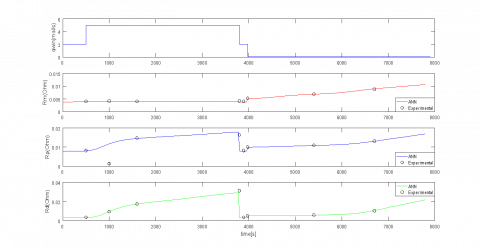Figure 8. Effect of membrane humidity change on parameters (Rm, Rp, Rd).

Table 3. Physical parameters of FC in a different operating state .

 Test Time[s] Rm[Ω] Rp[Ω] Rd[Ω] 1(Normal state) 500 0.00398 0.0080 0.0034 2 1000 0.00406 0.00123 0.0094 3 1600 0.00400 0.0147 0.0172 4 3800 0.00416 0.0163 0.0312 5 3980 0.00512 0.0099 0.0051 6 5400 0.00685 0.0108 0.0056 7 6700 0.00880 0.0130 0.0101

*Temperature constant and slowly increasing current(around 0.35 [A]).

4. Results and Discussion

Through what we observed in Figure 3, we set the value of qwin in three stages, to study the effect of RH % on the state of the fuel cell. As can be seen from Figure 8, qwin is held constant at 2 mol/s, between the interval 0 to 500 s. In this case, the fuel cell is in normal operating conditions (T and λ remain constant in all cases). But, between the interval 500 to 3800 s, the value of qwin is amounted to 5 mol/s. Hence, the fuel cell is in a flooding state. After that, the value of qwin drops to 0.2 mol/s, and thereby the fuel cell is in a dry state.

By comparing the attained results using the neural network model during deferent operating conditions (see Figure 8), we notice the follwing:

- In the normal phase [0, 500] s, the values of Rm, Rp, and Rd are constant, which means stability in the system.

- Between the two moments 500 s and 3800 s, it is observed that the value of Rm remains constant throughout the experiment period; on the contrary concerning Rp and Rd, we notice that their values increase by a large percentage in a short time due to the increasing of humidity inside the fuel cell.

- To find the effect of drought on the properties of the fuel cell, the value of qwin has been reduced to 0.2 mol/s. During this phase the value of Rm increases gradually; this refers to the drought of the fuel cell. While, the angle of inclination of the Rp and Rd curves in this case is smaller than the state of drowning, this also indicates in fuel cell drying state.

In Figure 9, we get the values Rm, Rp, and Rd utilising the neural network model for both drowning and dehydration after 3000 s. From this figure, it is easy to see that the value of Rm remains constant in the normal and drowning state, while it increases by 20% in the state of drying. Also, it is found that the value of polarization resistance increases by 25% and 40% in the case of drying and drowning, respectively, in comparison with to the normal state of fuel cell.

The difference in the values of Rd is caused by the difference in the area S due to the accumulation of water inside PEMFC.

## 9.jpg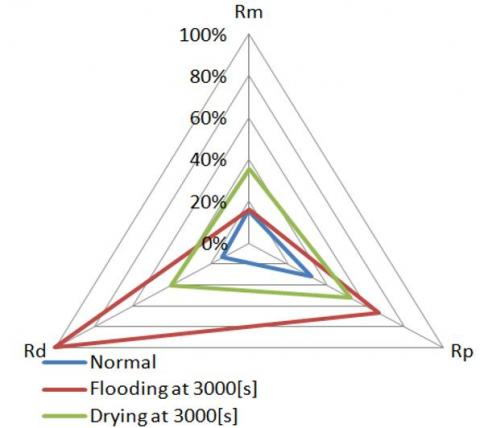Figure 9. Radar diagram for classification of the state of health in different operating conditions.

Figure 10 illustrates the values of Rm, Rd, and Rp in three-dimensional space. It appears that there are three regions: region A (between 0 s and 500 s) represents the normal state, region B (between 2000 s and 3800 s) refers to the case of the flood, and region C (between 5800 s and 6800 s) indicates that state of fuel cell is drought. Depending on these differences, we can estimate the state of health for the fuel cell.

## 1.png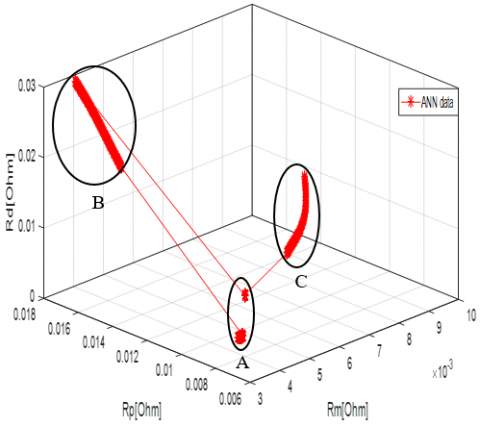Figure 10. 3D simulation of changing the fuel cell state during different time periods.

5. Conclusions

In this research, a neural network technology is used to determine the parameters of the electrochemical impedance, to estimate and diagnose the state of health of the fuel cell. Based on the achieved results, we found that there are three parameters that help to identify the state of PEMFC, which are Rm, Rp, and Rd (as shown in the Randles model). Also, this technology contributes to good diagnosis without the need for expensive equipment and therefore it is suitable for practical applications. Overall, our model can be adopted in the control system to better water flow rate management in both cases (i.e. flooding and drying) of fuel cell.

Nomenclature
 Vcel cell voltage (V) Ernest thermodynamic potential (V) Vact activation overvoltage (V) Vcon concentration overvoltage (V) VOhmic ohmic overvoltage (V) T Temperature (K) Jn Current density qH2in hydrogen inlet flow rates (mol/s) qO2in oxygen inlet flow rates (mol/s) qH2r hydrogen usage flow rates (mol/s) qO2r oxygen usage flow rates (mol/s) qwr water production flow rates (mol/s) CO2 oxygen concentration in the cathode active layer (mol.m−3) D diffusion coefficient F Faraday constant (A s mol−1) N number of electrons R perfect gas constant (J mol−1 K−1) Rd electrical resistance (Ω) Rm membrane resistance (Ω) Rp polarisation resistance (Ω) Q parameter of the CPE S active area (m²) tm membrane thickness (m) Z fuel cell impedance (Ω) ZCPE CPE impedance Zw Warburg impedance (Ω)

Greek Letters

 λa stoichiometry of air α power of the CPE τd the time constant of diffusion (s) ω pulsation (rad s−1) δ diffusion layer width (m) ξi parametric coefficient λ water membrane content σm membrane conductivity (Ω /cm) φ relative humidity (%) δ diffusion layer width (m)
References

 I. Sadli, “Modélisation par impédance d ’ une pile à combustible PEM pour utilisation en électronique de puissance Idris Sadli To cite this version : HAL Id : tel-01752782 soutenance et mis à disposition de l â€TM ensemble de la Contact : ddoc-theses-contact@univ-lorra,” 2018.

 N. A. Ahmed, M. Miyatake, and A. K. Al-Othman, “Power fluctuations suppression of stand-alone hybrid generation combining solar photovoltaic/wind turbine and fuel cell systems,” Energy Convers. Manag., vol. 49, no. 10, pp. 2711–2719, 2008, doi: 10.1016/j.enconman.2008.04.005.

 Y. Wang, K. S. Chen, J. Mishler, S. C. Cho, and X. C. Adroher, “A review of polymer electrolyte membrane fuel cells: Technology, applications, and needs on fundamental research,” Appl. Energy, vol. 88, no. 4, pp. 981–1007, 2011, doi: 10.1016/j.apenergy.2010.09.030.

 R. Petrone et al., “A review on model-based diagnosis methodologies for PEMFCs,” Int. J. Hydrogen Energy, vol. 38, no. 17, pp. 7077–7091, 2013, doi: 10.1016/j.ijhydene.2013.03.106.

 Z. Zheng et al., “A review on non-model based diagnosis methodologies for PEM fuel cell stacks and systems,” Int. J. Hydrogen Energy, vol. 38, no. 21, pp. 8914–8926, 2013, doi: 10.1016/j.ijhydene.2013.04.007.

 J. Wu, X. Zi Yuan, H. Wang, M. Blanco, J. J. Martin, and J. Zhang, “Diagnostic tools in PEM fuel cell research: Part II. Physical/chemical methods,” Int. J. Hydrogen Energy, vol. 33, no. 6, pp. 1747–1757, 2008, doi: 10.1016/j.ijhydene.2008.01.020.

 J. Wu, X. Z. Yuan, H. Wang, M. Blanco, J. J. Martin, and J. Zhang, “Diagnostic tools in PEM fuel cell research: Part I Electrochemical techniques,” Int. J. Hydrogen Energy, vol. 33, no. 6, pp. 1735–1746, 2008, doi: 10.1016/j.ijhydene.2008.01.013.

 F. Barbir, H. Gorgun, and X. Wang, “Relationship between pressure drop and cell resistance as a diagnostic tool for PEM fuel cells,” J. Power Sources, vol. 141, no. 1, pp. 96–101, 2005, doi: 10.1016/j.jpowsour.2004.08.055.

 R. T. White et al., “Four-dimensional joint visualization of electrode degradation and liquid water distribution inside operating polymer electrolyte fuel cells,” Sci. Rep., vol. 9, no. 1, pp. 1–12, 2019, doi: 10.1038/s41598-018-38464-9.

 N. Yousfi Steiner, D. Hissel, P. Moçtéguy, and D. Candusso, “Diagnosis of polymer electrolyte fuel cells failure modes (flooding & drying out) by neural networks modeling,” Int. J. Hydrogen Energy, vol. 36, no. 4, pp. 3067–3075, 2011, doi: 10.1016/j.ijhydene.2010.10.077.

 W. Mérida, D. A. Harrington, J. M. Le Canut, and G. McLean, “Characterisation of proton exchange membrane fuel cell (PEMFC) failures via electrochemical impedance spectroscopy,” J. Power Sources, vol. 161, no. 1, pp. 264–274, 2006, doi: 10.1016/j.jpowsour.2006.03.067.

 F. Z. Arama, K. Mammar, S. Laribi, A. Necaibia, and T. Ghaitaoui, “Implementation of sensor based on neural networks technique to predict the PEM fuel cell hydration state,” J. Energy Storage, vol. 27, no. May 2019, p. 101051, 2020, doi: 10.1016/j.est.2019.101051.

 O. Bethoux, M. Hilairet, and T. Azib, “A new on-line state-of-health monitoring technique dedicated to PEM fuel cell,” IECON Proc. (Industrial Electron. Conf., pp. 2745–2750, 2009, doi: 10.1109/IECON.2009.5415422.

 A. Saadi, M. Becherif, D. Hissel, and H. S. Ramadan, “Dynamic modeling and experimental analysis of PEMFCs: A comparative study,” Int. J. Hydrogen Energy, vol. 42, no. 2, pp. 1544–1557, 2017, doi: 10.1016/j.ijhydene.2016.07.180.

 N. Fouquet, C. Doulet, C. Nouillant, G. Dauphin-Tanguy, and B. Ould-Bouamama, "Model-based PEM fuel cell state-of-health monitoring via ac impedance measurements," J. Power Sources, vol. 159, no. 2, pp. 905–913, 2006, doi: 10.1016/j.jpowsour.2005.11.035.

 J. Mitzel et al., “Fault Diagnostics in PEMFC Stacks by Evaluation of Local Performance and Cell Impedance Analysis,” Fuel Cells, no. 0, pp. 1–10, 2020, doi: 10.1002/fuce.201900193.

 H. Lu, J. Chen, C. Yan, and H. Liu, “On-line fault diagnosis for proton exchange membrane fuel cells based on a fast electrochemical impedance spectroscopy measurement,” J. Power Sources, vol. 430, no. May, pp. 233–243, 2019, doi: 10.1016/j.jpowsour.2019.05.028.

 H. Nara, T. Yokoshima, and T. Osaka, “Technology of electrochemical impedance spectroscopy for an energy-sustainable society,” Curr. Opin. Electrochem., vol. 20, pp. 66–77, 2020, doi: 10.1016/j.coelec.2020.02.026.

 S. Jemeï, D. Hissel, M. C. Péra, and J. M. Kauffmann, "On-board fuel cell power supply modeling based on neural network methodology," J. Power Sources, vol. 124, no. 2, pp. 479–486, 2003, doi: 10.1016/S0378-7753(03)00799-7.

 C. Cadet, S. Jemeï, F. Druart, and D. Hissel, “Diagnostic tools for PEMFCs: From conception to implementation,” Int. J. Hydrogen Energy, vol. 39, no. 20, pp. 10613–10626, 2014, doi: 10.1016/j.ijhydene.2014.04.163.

 J. Kim, I. Lee, Y. Tak, and B. H. Cho, “State-of-health diagnosis based on hamming neural network using output voltage pattern recognition for a PEM fuel cell,” Int. J. Hydrogen Energy, vol. 37, no. 5, pp. 4280–4289, 2012, doi: 10.1016/j.ijhydene.2011.11.092.

 M. Hatti and M. Tioursi, “Dynamic neural network controller model of PEM fuel cell system,” Int. J. Hydrogen Energy, vol. 34, no. 11, pp. 5015–5021, 2009, doi: 10.1016/j.ijhydene.2008.12.094.

 M. Shao, X. J. Zhu, H. F. Cao, and H. F. Shen, "An artificial neural network ensemble method for fault diagnosis of proton exchange membrane fuel cell system," Energy, vol. 67, pp. 268–275, 2014, doi: 10.1016/j.energy.2014.01.079.

 G. N. Srinivasulu, T. Subrahmanyam, and V. D. Rao, “Parametric sensitivity analysis of PEM fuel cell electrochemical Model,” Int. J. Hydrogen Energy, vol. 36, no. 22, pp. 14838–14844, 2011, doi: 10.1016/j.ijhydene.2011.03.040.

 K. Mammar, F. Saadaoui, and A. Hazzab, “Development and simulation of a PEM fuel cell model for prediction of water content and power generation,” vol. 4, no. 2017, pp. 289–299, 2018, doi: 10.22104/ijhfc.2018.2792.1168.

 N. Fouquet, “Real-Time Model-Based Monitoring of a PEM Fuel Cell Flooding And Drying Out," pp. 0–7, 2010.

 K. Mammar, F. Saadaoui, and S. Laribi, “Design of a PEM fuel cell model for flooding and drying diagnosis using fuzzy logic clustering,” Renew. Energy Focus, vol. 30, no. 0, pp. 123–130, 2019, doi: 10.1016/j.ref.2019.06.001.

 B. A. Mccain, “Modeling and Analysis for Control of Reactant and Water Distributions in Fuel Cells by,” 2008.

 B. Wahdame et al., "Analysis of a PEMFC durability test under low humidity conditions and stack behavior modeling using experimental design techniques," J. Power Sources, vol. 182, no. 2, pp. 429–440, 2008, doi: 10.1016/j.jpowsour.2007.12.122.

 Laribi, S., Mammar, K., Sahli, Y., & Koussa, K. (2019). Analysis and diagnosis of PEM fuel cell failure modes (flooding & drying) across the physical parameters of electrochemical impedance model: Using neural networks method. Sustainable Energy Technologies and Assessments, 34, 35-42., doi: 10.1016/j.seta.2019.04.004.

 Amphlett, J. C., Baumert, R. M., Mann, R. F., Peppley, B. A., Roberge, P. R., & Rodrigues, A. (1994). Parametric modelling of the performance of a 5-kW proton-exchange membrane fuel cell stack. Journal of Power Sources, 49(1-3), 349-356..

 Boulon, L., Agbossou, K., Hissel, D., Hernandez, A., Bouscayrol, A., Sicard, P., & Péra, M. C. (2010, July). Energy management of a fuel cell system: Influence of the air supply control on the water issues. In 2010 IEEE International Symposium on Industrial Electronics (pp. 161-166). IEEE. doi: 10.1109/ISIE.2010.5637604.

 Agbossou, K., Dubé, Y., Hassanaly, N., Adzakpa, K. P., & Ramousse, J. (2009). Experimental validation of a state model for PEMFC auxiliary control. IEEE Transactions on Industry Applications, 45(6), 2098-2103. doi: 10.1109/TIA.2009.2031792.

 Wahdame, B., Candusso, D., Harel, F., François, X., Péra, M. C., Hissel, D., & Kauffmann, J. M. (2008). Analysis of a PEMFC durability test under low humidity conditions and stack behaviour modelling using experimental design techniques. Journal of Power Sources, 182(2), 429-440. doi: 10.1016/j.seta.2019.04.004.

 Halvorsen, I. J., Pivac, I., Bezmalinović, D., Barbir, F., & Zenith, F. (2020). Electrochemical low-frequency impedance spectroscopy algorithm for diagnostics of PEM fuel cell degradation. International Journal of Hydrogen Energy, 45(2), 1325-1334. doi: 10.1016/j.ijhydene.2019.04.004.

 Chevalier, S., Trichet, D., Auvity, B., Olivier, J. C., Josset, C., & Machmoum, M. (2013). Multiphysics DC and AC models of a PEMFC for the detection of degraded cell parameters. International Journal of Hydrogen Energy, 38(26), 11609-11618. doi: 10.1016/j.ijhydene.2013.04.057.